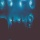Home Communities
IT Knowledge
Inspiration
Languages
EN

# Python - parse string to int

0 points
Created by:Lennox-A
515

In this article, we would like to show you how to parse the string to int in Python.

Quick solution:

``````text = "10"
print(int(text))  # 10``````

or

``````text = "10.99"

print(int(float(text)))  # 10
print(int(text))         # ValueError``````

## Practical example

In this example, we present how to parse string to int in two different cases.

1. When we have int number inside the string

``````text = "10"
print(int(text))  # 10``````

Output:

``10``

2. When we have float number inside the string

``````text1 = "10.01"
text2 = "10.99"

print(int(float(text1)))  # 10
print(int(float(text2)))  # 10``````

Output:

``````10
10``````
Join to our subscribers to be up to date with content, news and offers.